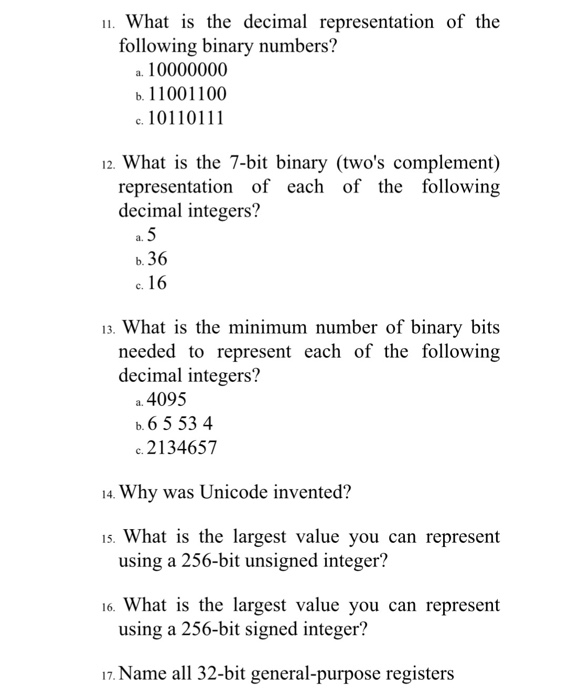# Question. What is the decimal representation of the following binary numbers? a. 10000000 b 1100 1100 с 101 101 1 1 12. What is the 7-bit binary (two’s complement) representation of each of the following decimal integers? b. 36 c. 13. What is the minimum number of binary bits needed